Your browser does not support JavaScript!

Search results

A Generalized multivariate skew-normal distribution with applications to spatial and regression predictions digital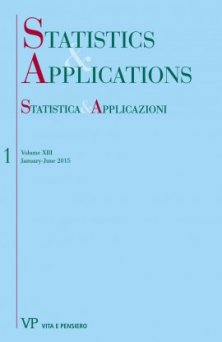format:Article| STATISTICA & APPLICAZIONI - 2015 - 1
Year: 2015
In this paper, a generalization to the multivariate skew-normal distribution of Arnold and Beaver (2002) is proposed. Also several distributional properties of the proposed distribution are explored. The proposed distribution has been used to define a stochastic process called the generalized-skew Gaussian process...
Regression model for proportions with probability masses at zero and one
Free
digital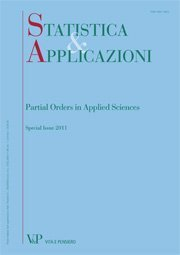format:Article| STATISTICA & APPLICAZIONI - 2012 - 2
Year: 2012
SUMMARY In many settings, the variable of interest is a proportion with high concentration of data at the boundaries. This paper proposes a regression model for a fractional variable with nontrivial probability masses at the extremes. In particular, the dependent variable is assumed to be a mixed random variable, obtained as the mixture of a Bernoulli and a beta random variables. The endpoints of zero and one are modelled by a logistic regression model. The values belonging to the interval (0,1) are assumed to be beta distributed and their mean and dispersion are jointly modelled by using two link functions. The regression model proposed here accommodates skewness and heteroscedastic errors. Finally, an application to loan recovery process of Italian banks is also provided. Keywords: Proportions, Mixed Random Variable, Beta Regression, Skewness, Heteroscedasticity.
Median ranked set sampling for polynomial regression
Free
digital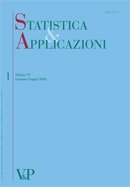format:Article| STATISTICA & APPLICAZIONI - 2011 - 1
Year: 2011
SUMMARY The purpose of this article is to study the ploynomial linear regression model under the median ranked set sampling (MRSS) scheme introduced by Muttlak (1997). If the response variable can be more easily ranked than quantified, then we use the MRSS to collect data by ranking on the response variable. We obtain estimators and confidence intervals for the polynomial regression parameters under MRSS when the errors have a symmetric distribution. We also show that the least square estimators, under MRSS, are more efficient than their SRS counterparts and give illustrating examples. Keywords: Median Ranked Set Sample, Polynomial Regression.
An integrated approach to regression analysis using correspondence analysis and cluster analysis
Free
digitalformat:Article| STATISTICA & APPLICAZIONI - 2010 - 1
Year: 2010
Problems involving dependent pairs of random variables usually involve two aspects: tests of independence or estimation of measures of association. In order to find out which way best explains the data, this paper addresses Regression Analysis applied to Correspondence Analysis (CA). It also uses Agglomerative Hierarchical Clustering as a method to accompany Multiple Correspondence Analysis (MCA). A well known data set is analyzed. Keywords: Complete Disjunctive Table, Burt Matrix, Regression Table, Multiple Correspondence Analysis, Agglomerative Hierarchical Clustering.
Moving extreme ranked set sampling for simple linear regression
Free
digitalformat:Article| STATISTICA & APPLICAZIONI - 2009 - 2
Year: 2009
The moving extreme ranked set sampling, introduced by Alodat and Al-Saleh (2001), is a modification of the well known ranked set sampling approach that was proposed by McIntyre (1952). In this paper, we suggest new estimators for the simple linear regression parameters under the moving extreme ranked set sampling scheme. Moreover, we show that the proposed estimators are more efficient than their counterparts using the simple random sampling approach. We illustrate our ideas and thoughts via simulation and data analysis and conduct a comparison between our approach and the traditional ones. Keywords: Moving ranked set sampling, Ranked set sampling, Simple linear regression.
A new OLS-based procedure for clusterwise linear regression
Free
digitalformat:Article| STATISTICA & APPLICAZIONI - 2009 - 1
Year: 2009
Data heterogeneity, within a (linear) regression framework, often suggest the use of a Clusterwise Linear Regression (CLR) procedure, which implies, among other things, the estimate of the appropriate number of clusters as well as the cluster membership of each unit. The approaches to the estimation of a CLR model are essentially based on the Ordinary Least Square (OLS) criterion or the likelihood criterion. In this paper, in a context of OLS approach, we propose an estimation of the model making use of an algorithm based on a threshold criterion for the determination coefficient of each cluster, to identify the appropriate number of clusters, and of a modified Spath’s algorithm, to estimate the cluster membership of each sample unit. A simulation design and an application to a real data-set show that the procedure outperforms other algorithms commonly used in literature.
The regression estimator in presence of ‘‘not at home’’
Free
digitalformat:Article| STATISTICA & APPLICAZIONI - 2008 - 1
Year: 2008
Being temporarily not at home can often cause the impossibility to give out a questionnaire to each selected unit of a sample; moreover, a high percentage of nonrespondents can strongly affect the quality of estimates. In order to solve this problem the ‘‘not at home’’ are usually called back until they become available; however, this methodology highly increases the costs of a survey. The main idea of this paper traces back to an estimation method early proposed by Politz and Simmons; in particular, a new estimator, based on the regression method, is proposed, so that the auxiliary information about the number of evenings spent at home by the units of the target population can be used. The proposed estimator is shown to be unbiased and more efficient than the one based only on the responses of the units being at home when first contacted. Moreover, unlike from the Politz-Simmons estimator, the variance of the proposed estimator can be easily determined and computed. Finally, in order to discuss the asymptotic properties of the regression estimator, the results of some simulations are reported; both the proposed estimator and the Politz-Simmons one turn out to be asymptotically unbiased; however, the regression estimator still proves to be more efficient.
Gini’s cograduation index for the estimation of the coefficients of a quadratic regression model digital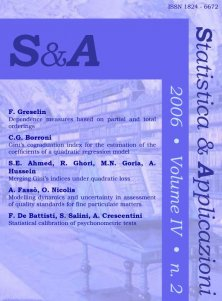format:Article| STATISTICA & APPLICAZIONI - 2006 - 2
Year: 2006
Least square estimates of regression parameters may become unreliable when some outliers affect the data. This fact forces to search for different methods of estimation, some of which consist of substituting ranks to observations to avoid influences by extremes values.
Un modello di regressione fuzzy per la valutazione della soddisfazione digital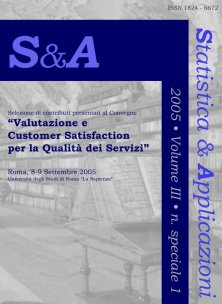format:Article| STATISTICA & APPLICAZIONI - 2005 - Special issue
Year: 2005
The paper considers the CS in its own nature of interval value and proposes to apply fuzzy regression models for the CS estimation.